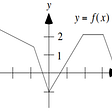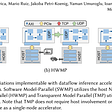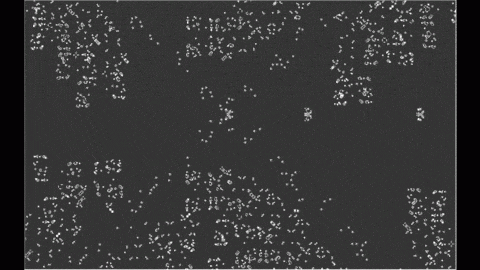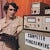# A short note on composition in TLA+

------ MODULE Queue ------EXTENDS Sequences, TLC, Integers, FiniteSetsCONSTANTS QueueIdRECURSIVE ReplaceInQueueDataInner(_,_,_,_)OUTQUEUE == 1OUT_I == 2LOCAL_DATA == 3TMPQ == 4TMPOUT == 5ReplaceInQueueDataInner(N,V,Val,Vec) ==    IF N = V    THEN <<Val>> \o Tail(Vec)    ELSE <<Vec>> \o ReplaceInQueueDataInner(N+1,V,Val,Tail(Vec))ReplaceInQueueData(V,Val,Vec) == ReplaceInQueueDataInner(1,V,Val,Vec)InitQueueState == << << >>, 0, << >>, {}, << >> >>\* incoming message format is <<cmd,sender,...>\* where cmd is\*\*  0 - erase my output\*  1 - enqueue for recipient(*--algorithm queuevariables  inqueue, \* ordered messages in  out = {}, \* repository for live  queue_data = InitQueueStateprocess QueueRunner = QueueIdbeginServiceInputs:    while TRUE doContinueQueueService:        print(222000);        await inqueue /= << >>;                print(222001);        queue_data := ReplaceInQueueData(LOCAL_DATA, Head(inqueue), queue_data);                print(222002);        inqueue := Tail(inqueue);        \* Remove output for this target.        if queue_data[LOCAL_DATA] = 0 then            print(222004);            out := out \ {(CHOOSE x \in out: x = queue_data[LOCAL_DATA])};        elseSendQueuedData:            print(222003);            print(queue_data[LOCAL_DATA]);            queue_data := ReplaceInQueueData(OUTQUEUE, Append(queue_data[OUTQUEUE], Tail(queue_data[LOCAL_DATA])), queue_data);        end if;                \* Try to place new outputsResetTmpq:        print(222005);        queue_data := ReplaceInQueueData(TMPQ, {}, queue_data);ResetOutI:        queue_data := ReplaceInQueueData(OUT_I, 1, queue_data);        StartDequeue:        while queue_data[OUT_I] <= Len(queue_data[OUTQUEUE]) doContinuqDequeue:            if {o \in out: o = queue_data[OUTQUEUE][queue_data[OUT_I]]} = {} then                queue_data := ReplaceInQueueData(TMPQ, queue_data[TMPQ] \union {queue_data[OUT_I]}, queue_data);                out := out \union {queue_data[OUTQUEUE][queue_data[OUT_I]]};            end if;IncOutI:            queue_data := ReplaceInQueueData(OUT_I, queue_data[OUT_I] + 1, queue_data);        end while;        print(222006);        \* Erase dequeued entries                queue_data := ReplaceInQueueData(TMPOUT, << >>, queue_data);ResetOutI2:        queue_data := ReplaceInQueueData(OUT_I, 1, queue_data);        StartFixup:        while queue_data[OUT_I] <= Len(queue_data[OUTQUEUE]) doContinueFixup:            if {i \in queue_data[TMPQ]: i = queue_data[OUT_I]} = {} then                queue_data := ReplaceInQueueData(TMPOUT, Append(queue_data[TMPOUT], queue_data[OUTQUEUE][queue_data[OUT_I]]), queue_data);            end if;IncOutI2:            queue_data := ReplaceInQueueData(OUT_I, queue_data[OUT_I] + 1, queue_data);        end while;        queue_data := ReplaceInQueueData(OUTQUEUE, queue_data[TMPOUT], queue_data);            end while;    end process;end algorithm;*)\*snip====
----- MODULE QueueProducer -----EXTENDS FiniteSets, Sequences, IntegersCONSTANT ProcessId, TargetId(*--algorithm producerprocess Producer = ProcessIdvariables    inqueue,    out = {}beginProduceAMessageForConsumer:    inqueue := Append(inqueue, <<1, TargetId, "hithere">>);end process;end algorithm; --*)\* snip=====
----- MODULE QueueConsumer -----EXTENDS TLC, Sequences, FiniteSetsCONSTANT ProcessId(*--algorithm consumerprocess Consumer = ProcessIdvariables    inqueue,    myitem,    out = {}beginAwaitQueueItemForMe:    print(444000);    await {x \in out: x = ProcessId} /= {};GotItemTakeIt:    print(444001);        myitem := CHOOSE x \in out: x = ProcessId;ShowItem:    print(myitem);RetireMyQueueOutput:    inqueue := Append(inqueue, <<0, ProcessId>>);end process;end algorithm;--*)\* snip=====
• Variables that are initialized to values other than our defaultInitValue must be preinitialized at the level above, otherwise initial setup will fail.
• The parent module ultimately instantiates _every_ piece of state used by each subset, so things like queue_data in Queue allow utilities to shorten that complexity to just one state variable and their formal inputs and outputs, but it all needs to be visible to the consumer.
• Since these runnable parts of the spec are distinct subsets of the state space, we’ll get messages like “Next is never enabled” from TLA+ if we don’t describe to TLA+ exactly what we want; that each INSTANCE’ Next rule is evaluated distinct from the others (\/ “or”) at the top level, and that each contains UNCHANGED rules for all the variables that belong to state it _isn’t_ involved in.
• pluscal uses a “pc” variable to drive the state machine. We must provide working space for it for each instance.
------ MODULE Test -------EXTENDS Naturals, TLC, SequencesVARIABLES inqueue, out, queue_pc, queue_data, producer_pc, consumer_pc, consumer_itemProducer == INSTANCE QueueProducer WITH ProcessId <- 2, TargetId <- 3, inqueue <- inqueue, out <- out, pc <- producer_pc, defaultInitValue <- << >>Consumer == INSTANCE QueueConsumer WITH ProcessId <- 3, pc <- consumer_pc, myitem <- consumer_item, defaultInitValue <- << >>Queue == INSTANCE Queue WITH QueueId <- 1, inqueue <- inqueue, out <- out, pc <- queue_pc, queue_data <- queue_data, defaultInitValue <- << >>Init == out = {} /\ Queue!Init /\ Producer!Init /\ Consumer!InitNext ==     \/ (Queue!Next /\ UNCHANGED << producer_pc, consumer_pc, consumer_item >>)     \/ (Producer!Next /\ UNCHANGED << queue_data, queue_pc, consumer_pc, consumer_item >>)    \/ (Consumer!Next /\ UNCHANGED << queue_data, queue_pc, producer_pc >>)=====

--

--

--

## More from art yerkes

An old programmer learning new tricks.

Love podcasts or audiobooks? Learn on the go with our new app.

## GMAT, GRE Functions and Graphing Question## dbt Orchestration in Azure DevOps Pipelines## Scaling Performance of DNN Inference in FPGA clusters using InAccel orchestrator## [Part 2] Delivering a Unified Omnichannel Experience using Headless Commerce## How low code helps software developers embrace rapid change## Conway’s Game of Life (in Ruby)## Another story about microservices: Hexagonal Architecture## art yerkes

An old programmer learning new tricks.

## “She Waved at Me”## Libraries on the margins## CS373 Spring 2022: Scarlett Shires, Week 2## Language and Culture: The Creolization of Sheng